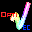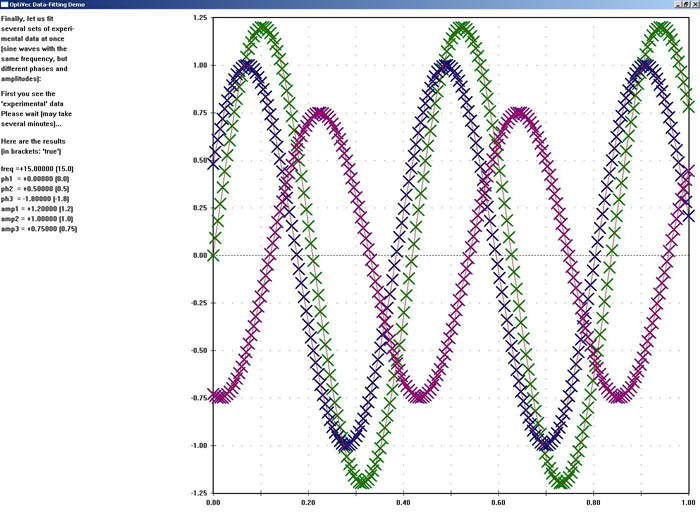# OptiVec for Linux GCC / CLang

8.0.1
OptiVec: Fast vector and matrix library for Linux with GCC or CLangFast vector and matrix library for Linux
OptiVec contains more than 3500 hand-optimized, Assembler-written functions for all floating-point and integer data types from the following fields: 1. Vectorized form of arithmetic operators and math functions. 2. Matrix operations, e.g.: multiplication, inversion, LU decomposition, singular value decomposition, eigenvalues. 3. Fast Fourier Transform techniques for efficient convolutions, correlation analyses, spectral filtering, etc., both one- and two-dimensional. 4. Curve fitting for a wide range of model functions from simple linear regression to non-linear models with multiple data sets. 5. Statistics. 6. Analysis (derivatives, integrals, extrema, interpolation). 7. Complex number math, both in cartesian and polar format. The vectorized implementation in Assembler makes OptiVec functions typically 3-5 times faster than compiled source code of the same functionality. In many instances, the numerical accuracy is improved as well. The object-oriented interface for C++, "VecObj", offers simplified function calls and increased memory safety. This version is for Linux with GCC or CLang.
Technical details
Title:
OptiVec for Linux GCC / CLang 8.0.1 for Linux
Requirements:
GCC or CLang on Linux
OS Support:
Linux, Linux Console, Linux Gnome, Linux GPL, Linux Open Source
Language:
German, English